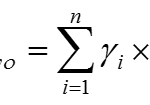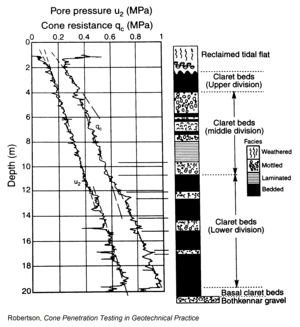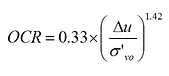Overburden stress, also called vertical stress or overburden pressure, is the pressure imposed on a layer of soil by the weight of the layers on top of it. Overburden stress can cause errors or drift in CPT measurements, creating the need for correction factors in deeper tests depths and soft or fine-grained soils. However, overburden stress is also useful in determining the soil’s mechanical properties. In this blog, we’ll give an overview of the effect of overburden stress on CPT testing and what we can learn from it.
The formula for overburden stress is given by:σvo = overburden stress
ɤi = in situ density of soil layer
hi = height of soil layerIf it’s been a while since you’ve seen summation notation, this means that for each soil layer, you multiply the density of the layer by its height, then add all the resulting weights together until the pressure at the desired depth is known. In practice, the exact height and density of the soil layers at the test site are usually not known, so you may have to determine an average density based on what you do know about the geology of the area.
CPT measurements of tip resistance, sleeve friction and pore pressure tend to increase along with increasing depth and increasing overburden stress. This effect can be seen in the graph at right. For this reason, we correct for overburden stress in calculating the normalized friction ratio and normalized tip resistance: to ensure that your data is consistent, it is important to use these parameters in deep tests and in soft, fine-grained soils, as we discussed in an earlier blog.
In addition to normalized CPT parameters, overburden pressure allows us to understand and calculate the following engineering parameters:

• Effective overburden stress: the effective stress on the soil skeleton, which is calculated by subtracting the pore pressure from the overburden stress
• Over consolidation ratio: the ratio of past maximum effective overburden stress to present effective overburden stress. (Consolidation refers to a process in which soil decreases in volume.) A soil that is currently under its maximum effective overburden stress is said to be normally consolidated and has an OCR of 1. A soil that once experienced a greater pressure (for example, if it was once under a glacier) is considered over consolidated and will have a higher OCR. This past maximum pressure is known as pre-consolidation pressure. (Note: OCR is defined differently for cemented or aged soils.)
In a CPT test, the OCR is commonly calculated based on the ratio of the excess pore pressure (∆u) and the overburden stress:∆u = excess pore pressure (the transient increase in pore pressure when the CPT cone is advanced)
σ’vo = effective overburden stress

Overburden stress is also important in determining the soil shear strength of the soil and the soil liquefaction potential. These topics deserve in-depth treatment, and we’ll cover them in a later blog.